• RU
•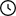Waiting For Moderation: 37

# Traction-dynamic calculation of a car with the development of a driving bridge - heading

• Size: 558 KB

## Description

Course project with the development of the MAZ-53362 leading bridge. Includes engine selection, calculation of external speed characteristic of the engine, calculation of transmission gear ratios, calculation of kinematic speed by gears, calculation of traction characteristic of the car, calculation of dynamic characteristic, calculation of acceleration dynamics, calculation of time and acceleration path, description of the structure device, strength calculation, as well as drawing of the bridge and graphics

## Project's Content_53362.rar [ 558 KB ]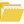МАЗ-53362, Вед мост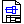Вн.скор.cdw [ 107 KB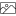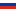]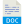ПЗ.doc [ 2 MB]Редуктор заднего моста МАЗ с колесным редуктором(разъемный).cdw [ 266 KB]Содержание.doc [ 39 KB]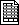Спецификация СБ редуктора.spw [ 66 KB]Титульный лист.doc [ 27 KB]

### Contents

Introduction

1 Engine selection

2 Calculation of engine external speed characteristic

3 Calculation of transmission gear ratios

4 Calculation of kinematic speed by gears

5 Calculation of the traction characteristic of the car

6 Dynamic characteristic calculation

7 Calculation of acceleration dynamics

8 Calculation of acceleration time and path

9 Description of the structure device

10 Strength calculation

Conclusion

Literature

Application

### Introduction

The main objective of the course project is the traction dynamic calculation of the MAZ-53362 car with the development of a driving axle, which includes the determination of engine and transmission characteristics that ensure the required traction and fuel efficiency of the car under given operating conditions.

The project solves the following problems for calculating the traction dynamic characteristics of a car with the development of a driving axle: engine selection (maximum power calculation), engine external speed characteristic, calculation of transmission ratios, calculation of the kinematic speed of the car by gears, car traction characteristic, car dynamic characteristic, car acceleration dynamics characteristic, power calculation of the driving axle.

### Conclusion

The course project was based on the calculation and design of the leading bridge, paying attention to a thorough study of the design in all its details.

The main task of traction calculation was to determine the characteristics of the engine and transmission, which provide the required traction speed characteristics, as well as the strength calculation of the driving bridge .

During the design process, the driving bridge of the MAZ-53362 prototype car was carefully considered and studied.

Drawings contentВн.скор.cdwРедуктор заднего моста МАЗ с колесным редуктором(разъемный).cdwСпецификация СБ редуктора.spw